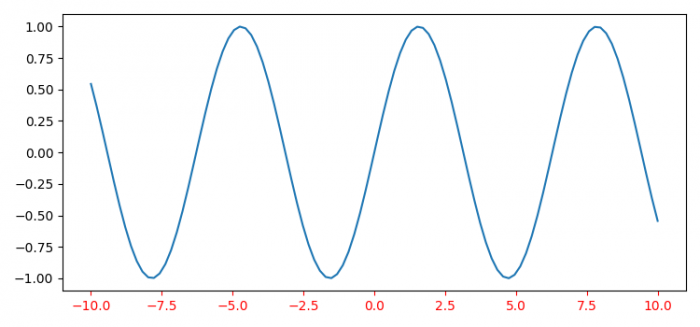# Changing the color of a single X-axis tick label in Matplotlib

To change the color of a single X-axis tick label in matplotlib, we can take the following steps −

• Set the figure size and adjust the padding between and around the subplots.
• Create a new figure or activate an existing figure.
• Add an '~.axes.Axes' to the figure as part of a subplot arrangement.
• Create x and y data points using numpy.
• Plot x and y data points using plot() method.
• To set the color of X-axis tick label in matplotlib, we can use tick_params() method with axis='x' and color='red'.
• To display the figure, use show() method.

## Example

import numpy as np
from matplotlib import pyplot as plt

plt.rcParams["figure.figsize"] = [7.50, 3.50]
plt.rcParams["figure.autolayout"] = True

fig = plt.figure()

x = np.linspace(-10, 10, 100)
y = np.sin(x)

plt.plot(x, y)

ax.tick_params(axis='x', colors='red')

plt.show()

## Output